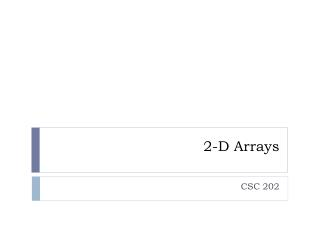Download Presentation2-D Arrays# 2-D Arrays - PowerPoint PPT Presentation

Download Presentation##### 2-D Arrays

Download Policy: Content on the Website is provided to you AS IS for your information and personal use and may not be sold / licensed / shared on other websites without getting consent from its author. While downloading, if for some reason you are not able to download a presentation, the publisher may have deleted the file from their server.

- - - - - - - - - - - - - - - - - - - - - - - - - - - E N D - - - - - - - - - - - - - - - - - - - - - - - - - - -
##### Presentation Transcript

1. 2-D Arrays CSC 202

2. A Brief Introduction to Two-Dimensional Arrays • An example of declaring a one-dimensional array that can contain 5 integers: • int [ ] example = new int ; • An example of declaring a two-dimensional array: • int [ ] [ ] twoD = new int  ; • This example creates a two-dimensional array that has 3 rows and 5 columns.

3. Filling a 2-D Array • So, if the array were filled with the value 9 in each position, it could be thought of as appearing like this in memory: 9 9 9 9 9 9 9 9 9 9 9 9 9 9 9

4. Filling a 2-D Array • Suppose you fill the array using these loops: • for ( inti=0, i < twoD.length, i++) for (int j=0, j < twoD [i].length, j++) twoD [i] [j] = i + j; • What would the array look like in memory?

5. A Program For You //M. M. Pickard 1/27/2011 /* This program declares a 2-D array, fills it with values, and displays its contents. It can be found at ftp://cobweb.sfasu.edu/csc/mpickard/ftp/CSC202/TwoD_ArrayExample.java */ public class TwoD_ArrayExample{ public static void main (String args[]){ int[ ] [ ] twoD = new int  ; // declares a table with three rows and five columns for ( inti=0; i < twoD.length; i++) // iterates over all rows for (int j=0; j < twoD [i].length; j++) // iterates over all columnstwoD [i] [j] = i + j; // inserts a value at the intersection of the ith row and the jth column for ( inti=0; i < twoD.length; i++){ // iterates over all rows for (int j=0; j < twoD [i].length; j++) // iterates over all columns System.out.print(twoD[i] [j] + " "); //displays an element found in the ith row and the jthcol System.out.println(); // goes to a new line at the end of each row } // end for } // end main } // end class

6. Important Concepts • Arrays of Arrays • Ragged Arrays • Multidimensional Arrays